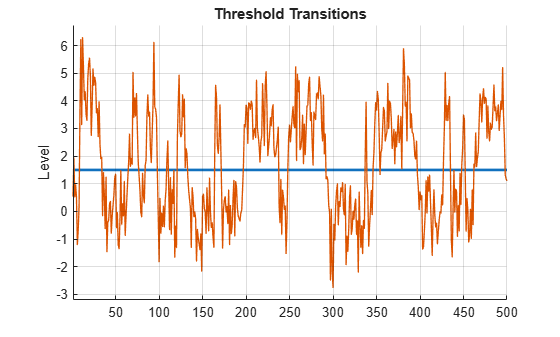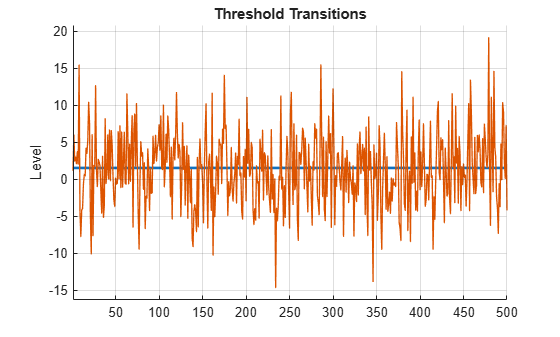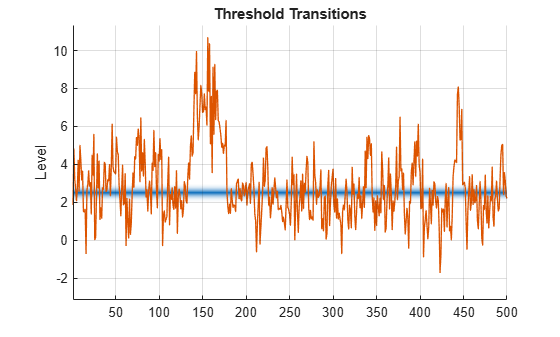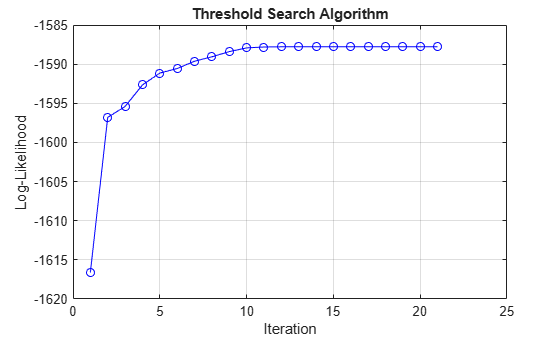# Estimate Threshold-Switching Dynamic Regression Models

This example shows how to use the `estimate` function to fit threshold-switching dynamic regression models to simulated data and to tune the estimation procedure. The example estimates a self-exciting threshold autoregressive model (SETAR), a TAR model with an exogenous threshold variable, and a self-exciting smooth TAR model (SESTAR) containing a regression component.

Threshold-switching dynamic regression model estimation, performed by `estimate`, is an iterative two-step procedure:

1. Conduct a constrained, nonlinear search over threshold transition levels and rates.

2. Solve a linear least-squares problem for submodel parameters.

The resulting estimates of parameter standard errors, data loglikelihood, and model residuals are conditional on the optimal threshold parameters computed by the search.

### Simulate Data from SETAR DGP

Consider a data-generating process (DGP) for the following simple univariate, mean-switching, SETAR model:

• The discrete, endogenous threshold transition is at mid-level 1.5.

• ${\sigma }^{2}=1$ is the model-wide process variance.

• The state 1 submodel is ${\mathit{y}}_{1,\mathit{t}}=0.2{\mathit{y}}_{1,\mathit{t}-1}+{\epsilon }_{1.\mathit{t}}$, where ${\epsilon }_{1,\mathit{t}}\sim Ν\left(0,{\sigma }^{2}\right)$.

• The state 2 submodel is ${\mathit{y}}_{2,\mathit{t}}=0.4{\mathit{y}}_{2,\mathit{t}-1}+{\epsilon }_{2.\mathit{t}}$, where ${\epsilon }_{2,\mathit{t}}\sim Ν\left(0,{\sigma }^{2}\right)$.

Create a SETAR model that represents the DGP by following this procedure:

1. Create the threshold transition by using the `threshold` function. The resulting `threshold` object represents all aspects of the threshold transition except for whether the associated threshold variable is endogenous or exogenous to the threshold-switching model system.

2. Create each univariate submodel by using the `arima` function. The resulting `arima` objects each characterize the dynamics of the system within their respective states. Concatenate the model objects in a vector.

3. Create the threshold-switching model by passing the threshold transitions object and state submodel vector to the `tsVAR` function. Specify the model-wide covariance by using the `Covariance` name-value argument. The resulting `tsVAR` object represents all aspects of the threshold-switching model for whether the associated threshold variable is endogenous or exogenous to its system. Object functions of the `tsVAR` object require knowledge of the threshold variable type (the default is endogenous or self-exciting for all object functions, with a delay of 1 time step).

```midlvl = 1.5; ttDGP = threshold(midlvl); mdl1DGP = arima(Constant=0,AR=0.2); mdl2DGP = arima(Constant=2,AR=0.4); MdlDGP = tsVAR(ttDGP,[mdl1DGP mdl2DGP],Covariance=1);```

`MdlDGP` is a `tsVAR` object that represents the DGP. It is fully specified because all parameters are set to known values.

Generate a random path of length 500 from the SETAR DGP by using the `simulate` object function. Plot the threshold transition and the path.

```seed = 0; rng(seed) % For reproducibility T = 500; % Sample size Y = simulate(MdlDGP,T); % SETAR path figure ttplot(ttDGP,Data=Y)```The plot shows the self-exciting response variable sampling states on either side of the threshold level. In practice, without knowledge of the DGP, a plot of empirical data in `Y` informs a reasonable choice of the initial threshold level, which is `estimate` requires.

### Create Model Template for Estimation

To estimate a SETAR model of the data, create the following variables:

• A model template for estimation, which is a partially specified `tsVAR` object that indicates the structure of the target model with `NaN` values for estimable parameters

• A threshold transition to initialize the search, which is a fully specified `threshold` object. This example uses `1` as the initial mid-level.

```tt = threshold(NaN); mdl1 = arima(Constant=NaN,AR=NaN); mdl2 = arima(Constant=NaN,AR=NaN); Mdl = tsVAR(tt,[mdl1 mdl2],Covariance=NaN); t0 = 1; % Initial mid-level tt0 = threshold(t0,Type="discrete");```

### Fit SETAR Model to Data

Discrete transitions pose a unique estimation challenge because they fail to disambiguate levels that fall between threshold variable data, which produces discontinuous objective functions that are difficult to optimize. To address this problem, `estimate` treats discrete transition levels as high-rate logistic transitions before it converts to discrete levels and discards the rates at estimation termination . The `MaxRate` name-value argument specifies the upper bound for the rates `estimate` uses for estimation.

This code shows the internal representation of the discrete transition during estimation by plotting a logistic threshold transition at mid-level 1.5 with rate 10.

```maxRate = 10; ttEst = threshold(midlvl,Type="logistic",Rates=maxRate); figure ttplot(ttEst,Data=Y) colorbar("off")```The logistic transition combines the two submodels in a weighted mixture model that is responsive to continuous changes in level during the threshold search procedure. `estimate` automatically implements the transformation from a discrete to a logistic representation at the beginning of estimation, and then it reverse-transforms the resulting threshold transition at estimation termination.

Estimate the SETAR model by passing the model template `Mdl`, initial threshold transition `tt0`, and simulated path `Y` to `estimate`. Set the maximum transition rate to `10`. By default, the threshold variable is endogenous (`Type="endogenous"`) with a delay of one time step (`Delay=1`).

`EstMdl = estimate(Mdl,tt0,Y,MaxRate=maxRate);`

`EstMdl` is a fully specified `tsVAR` object representing the estimated SETAR model with a discrete threshold transition. `estimate` uses the maximum rate `maxRate` only internally because it represents the discrete transition as logistic to overcome numerical problems during optimization. To tune the estimation procedure for any threshold transition type, you can iterate by adjusting `MaxRate` and calling `estimate`.

### Inspect Estimation Results

Display the estimated threshold transition `Switch`, stored in the estimated model object, by using dot notation.

`EstTT = EstMdl.Switch`
```EstTT = threshold with properties: Type: 'discrete' Levels: 1.5233 Rates: [] StateNames: ["1" "2"] NumStates: 2 ```

Display the estimated submodels.

`EstMdl.Submodels`
```ans = 2x1 varm array with properties: NumSeries P Constant AR Beta Trend Covariance SeriesNames Description ```

Observe that `estimate` returns a vector of `varm` model objects representing the estimated univariate models, although the model template `Mdl` specifies a vector of `arima` model objects.

Visually compare the estimated parameters to those of the DGP.

```EstMdlParams = [EstMdl.Switch.Levels; ... EstMdl.Submodels(1).Constant; ... EstMdl.Submodels(2).Constant; ... EstMdl.Submodels(1).AR{:}; ... EstMdl.Submodels(2).AR{:}; ... EstMdl.Covariance]; DGPParams = [MdlDGP.Switch.Levels; ... MdlDGP.Submodels(1).Constant; ... MdlDGP.Submodels(2).Constant; ... MdlDGP.Submodels(1).AR{:}; ... MdlDGP.Submodels(2).AR{:}; ... MdlDGP.Covariance]; table(DGPParams,EstMdlParams)```
```ans=6×2 table DGPParams EstMdlParams _________ ____________ 1.5 1.5233 0 0.034171 2 1.8688 0.2 0.14564 0.4 0.41827 1 1.0406 ```

The estimation results, with sufficient sample size and a limited number of parameters, approximate values of the DGP.

Summarize the estimation results.

`summarize(EstMdl)`
```Description 1-Dimensional tsVAR Model with 2 Submodels Switch Transition Type: discrete Estimated Levels: 1.523 Fit Effective Sample Size: 499 Number of Estimated Parameters: 4 Number of Constrained Parameters: 0 LogLikelihood: -717.972 AIC: 1443.945 BIC: 1460.795 Submodels Estimate StandardError TStatistic PValue ________ _____________ __________ __________ State 1 Constant(1) 0.034171 0.067052 0.50963 0.61031 State 1 AR{1}(1,1) 0.14564 0.077043 1.8904 0.0587 State 2 Constant(1) 1.8688 0.22499 8.3063 9.8696e-17 State 2 AR{1}(1,1) 0.41827 0.064622 6.4726 9.6348e-11 ```

### Fit TAR Model with Exogenous Threshold Variable

The estimation of AR parameters in a self-exciting model is complicated by the mixed endogenous dynamics.

Compare SETAR model estimation to estimation of a TAR model, with the same general structure as the SETAR model, but with an exogenous threshold variable. Consider the threshold variable ${\mathit{z}}_{\mathit{t}}=1.5+5{\mathit{u}}_{\mathit{t}}$, where ${\mathit{u}}_{\mathit{t}}$ is standard Gaussian noise.

Simulate a random path of threshold variable observations, and then plot the threshold transition with the exogenous threshold data.

```z = 1.5 + 5*randn(T,1); % Path of exogenous threshold data figure ttplot(ttDGP,Data=z)```As expected, ${\mathit{z}}_{\mathit{t}}$ randomly fluctuates around the threshold.

Simulate a response path from the DGP of the TAR model. Specify that the threshold variable is exogenous and specify the simulated threshold data.

```rng(seed) Y = simulate(MdlDGP,T,Type="exogenous",Z=z); % TAR path```

Estimate the TAR model with an exogenous threshold variable. Threshold variable aside, the structure of the SETAR and TAR models are identical. Therefore, specify the SETAR model template and initial threshold transition. Specify that the threshold variable is exogenous and specify the simulated threshold data and the maximum transition rate `maxRate`.

```EstMdlExo = estimate(Mdl,tt0,Y,MaxRate=maxRate, ... Type="exogenous",Z=z);```

Visually compare the estimated parameters to those of the DGP.

```EstMdlExoParams = [EstMdlExo.Switch.Levels; ... EstMdlExo.Submodels(1).Constant; ... EstMdlExo.Submodels(2).Constant; ... EstMdlExo.Submodels(1).AR{:}; ... EstMdlExo.Submodels(2).AR{:}; ... EstMdlExo.Covariance]; table(DGPParams,EstMdlParams,EstMdlExoParams)```
```ans=6×3 table DGPParams EstMdlParams EstMdlExoParams _________ ____________ _______________ 1.5 1.5233 1.5391 0 0.034171 0.0048078 2 1.8688 1.9463 0.2 0.14564 0.18852 0.4 0.41827 0.41886 1 1.0406 1.0216 ```

Overall, the estimates improve with the exogenous threshold data.

Summarize the estimation results.

`summarize(EstMdlExo)`
```Description 1-Dimensional tsVAR Model with 2 Submodels Switch Transition Type: discrete Estimated Levels: 1.539 Fit Effective Sample Size: 499 Number of Estimated Parameters: 4 Number of Constrained Parameters: 0 LogLikelihood: -713.386 AIC: 1434.773 BIC: 1451.623 Submodels Estimate StandardError TStatistic PValue _________ _____________ __________ ___________ State 1 Constant(1) 0.0048078 0.08187 0.058726 0.95317 State 1 AR{1}(1,1) 0.18852 0.039812 4.7352 2.1887e-06 State 2 Constant(1) 1.9463 0.087457 22.254 1.0253e-109 State 2 AR{1}(1,1) 0.41886 0.040425 10.361 3.7112e-25 ```

### Tune Estimation Procedure

The `MaxLevel` and `MaxRate` name-value arguments set inequality constraints on the threshold parameter search. Estimate the TAR model with an exogenous threshold variable again. Expand the search domain by increasing the upper bound of the rate.

```maxRate = 50; EstMdlExo2 = estimate(Mdl,tt0,Y,MaxRate=maxRate,Type="exogenous",Z=z);```

Compare the results of the two `MaxRate` settings for the TAR model esimtation.

```EstMdlExoParams2 = [EstMdlExo2.Switch.Levels; ... EstMdlExo2.Submodels(1).Constant; ... EstMdlExo2.Submodels(2).Constant; ... EstMdlExo2.Submodels(1).AR{:}; ... EstMdlExo2.Submodels(2).AR{:}; ... EstMdlExo2.Covariance]; table(DGPParams,EstMdlParams,EstMdlExoParams2)```
```ans=6×3 table DGPParams EstMdlParams EstMdlExoParams2 _________ ____________ ________________ 1.5 1.5233 1.5185 0 0.034171 0.0066017 2 1.8688 1.9409 0.2 0.14564 0.18838 0.4 0.41827 0.41627 1 1.0406 1.0063 ```

In this case, the expanded search domain results in more accurate estimates.

### Fit SESTAR Model Containing Regression Component

Accurate estimation is more challenging when the number of series, thresholds, and submodels increases for the following reasons:

• The estimates are asymptotic.

• When the estimation procedure estimates submodel parameters, the demand on the sample size increases because the procedure divides the sample into subsamples through transition-function weighting.

Consider a DGP for a bivariate, two-state, self-exciting, smooth TAR model (SESTAR) with both endogenous and exogenous dynamics:

• The smooth, normal threshold transition is at mid-level 2.5 with a transition rate of 5.

• The state 1 submodel is ${\mathit{y}}_{1,\mathit{t}}=\left[\begin{array}{c}1\\ -1\end{array}\right]+\left[\begin{array}{cc}0.5& 0\\ 0& 0.5\end{array}\right]{\mathit{y}}_{1,\mathit{t}-1}+\left[\begin{array}{c}0.3\\ -0.3\end{array}\right]{\mathit{x}}_{1,\mathit{t}}+{\epsilon }_{1.\mathit{t}}$.

• The state 2 submodel is ${\mathit{y}}_{2,\mathit{t}}=\left[\begin{array}{c}2\\ -2\end{array}\right]+\left[\begin{array}{cc}0.3& 0\\ 0& 0.3\end{array}\right]{\mathit{y}}_{2,\mathit{t}-1}+\left[\begin{array}{cc}0.4& 0\\ 0& 0.4\end{array}\right]{\mathit{y}}_{2,\mathit{t}-2}+\left[\begin{array}{cc}0.5& 0.2\\ -0.5& -0.2\end{array}\right]\left[\begin{array}{c}{\mathit{x}}_{1,\mathit{t}}\\ {\mathit{x}}_{2,\mathit{t}}\end{array}\right]+{\epsilon }_{2.\mathit{t}}$.

• ${\mathit{x}}_{1,\mathit{t}}$ and ${\mathit{x}}_{2,\mathit{t}}$ are univariate, standard Gaussian exogenous series.

• ${\epsilon }_{1,\mathit{t}}\sim {Ν}_{2}\left(\left[\begin{array}{c}0\\ 0\end{array}\right],\left[\begin{array}{cc}1& 0\\ 0& 1\end{array}\right]\right)$ and ${\epsilon }_{2.\mathit{t}}={\epsilon }_{1,\mathit{t}}\sqrt{2}$.

Create a SETAR model that represents the DGP by following this procedure. Use `varm` to specify the submodel dynamics.

```ttDGP = threshold(2.5,Type="normal",Rates=5); % Constants (numSeries x 1 vectors) c1 = [1;-1]; c2 = [2;-2]; % AR coefficients (numSeries x numSeries matrices) AR1 = {[0.5 0; 0 0.5]}; % 1 lag AR2 = {[0.3 0; 0 0.3] [0.4 0; 0 0.4]}; % 2 lags % Regression coefficients (numSeries x numRegressors matrices) beta1 = [0.3; -0.3]; % 1 regressor Beta2 = [0.5 0.2; -0.5 -0.2]; % 2 regressors % Innovations covariances (numSeries x numSeries matrices) Sigma1 = [1 0; 0 1]; Sigma2 = [2 0; 0 2]; mdl1DGP = varm(Constant=c1,AR=AR1,Beta=beta1,Covariance=Sigma1); mdl2DGP = varm(Constant=c2,AR=AR2,Beta=Beta2,Covariance=Sigma2); MdlDGP = tsVAR(ttDGP,[mdl1DGP mdl2DGP]);```

Simulate a 2-D path of standard Gaussian values for the regression data, and then simulate a 2-D response path from the DGP. By default, `tsVAR` object functions set the threshold variable of a multivariate self-exciting model to the first variable (`Index=1`).

```rng(seed) X = rand(T,2); % Regression data Y = simulate(MdlDGP,T,X=X); % SESTAR path figure ttplot(ttDGP,Data=Y(:,1)) % Self-exciting component of Y colorbar('off')```The plot indicates that the self-exciting first component of the response samples states on either side of the threshold level.

#### Unconstrained Estimation

Estimate the two threshold parameters and all 22 constant, AR, and regression submodel parameters from the simulated sample. To show the progress of the search procedure, create an iteration plot by using the `IterationPlot` name-value argument.

```tt = threshold(NaN,Type="normal",Rates=NaN); mdl1 = varm(Constant=NaN(2,1),AR={NaN(2)},Beta=NaN(2,1)); mdl2 = varm(Constant=NaN(2,1),AR={NaN(2) NaN(2)},Beta=NaN(2,2)); Mdl = tsVAR(tt,[mdl1 mdl2]); figure tt0 = threshold(1,Type="normal",Rates=1); EstMdlSESTAR = estimate(Mdl,tt0,Y,X=X,IterationPlot=true);```The plot indicates convergence after 20 iterations.

Compare the DGP parameters and estimated values.

`compareEstimates(MdlDGP,EstMdlSESTAR)`
```Threshold parameters: 2.5000 2.6484 5.0000 4.6065 Submodel constants: 1.0000 1.4785 -1.0000 -1.2181 2.0000 1.0724 -2.0000 -1.7798 Submodel(1) AR coefficients: 0.5000 0 0.4550 0.0710 0 0.5000 0.0482 0.4903 Submodel(2) AR coefficients: 0.3000 0 0.3327 -0.1410 0 0.3000 -0.1514 0.2909 0.4000 0 0.3539 0.4015 0 0.4000 0.5119 0.3694 Submodel(1) regression coefficients: 0.3000 -0.0039 -0.3000 -0.0400 Submodel(2) regression coefficients: 0.5000 0.2000 0.3600 0.2931 -0.5000 -0.2000 -0.4952 0.2515 Innovations covariances: 1.0000 0 1.1646 0.0744 0 1.0000 0.0744 0.9572 2.0000 0 1.8074 0.1777 0 2.0000 0.1777 1.8293 ```

In addition to the large number of parameters to estimate, the estimation procedure can struggle to distinguish the mix of self-exciting, AR, and exogenous dynamics. The results show a range of accuracies relative to the DGP, depending on the parameter.

Summarize the estimation results:

`summarize(EstMdlSESTAR)`
```Description 2-Dimensional tsVAR Model with 2 Submodels Switch Transition Type: normal Estimated Levels: 2.648 Estimated Rates: 4.607 Fit Effective Sample Size: 498 Number of Estimated Parameters: 22 Number of Constrained Parameters: 0 LogLikelihood: -1587.781 AIC: 3219.561 BIC: 3312.195 Submodels Estimate StandardError TStatistic PValue __________ _____________ __________ __________ State 1 Constant(1) 1.4785 0.25733 5.7455 9.1639e-09 State 1 Constant(2) -1.2181 0.24873 -4.8972 9.7196e-07 State 1 AR{1}(1,1) 0.45497 0.098613 4.6137 3.9548e-06 State 1 AR{1}(2,1) 0.048204 0.095317 0.50573 0.61305 State 1 AR{1}(1,2) 0.071037 0.060538 1.1734 0.24062 State 1 AR{1}(2,2) 0.49026 0.058515 8.3784 5.3629e-17 State 1 Beta(1,1) -0.0039272 0.27741 -0.014157 0.98871 State 1 Beta(2,1) -0.039972 0.26814 -0.14907 0.8815 State 2 Constant(1) 1.0724 0.40609 2.6408 0.0082704 State 2 Constant(2) -1.7798 0.39252 -4.5344 5.7775e-06 State 2 AR{1}(1,1) 0.33272 0.073856 4.505 6.6377e-06 State 2 AR{1}(2,1) -0.15145 0.071387 -2.1215 0.033883 State 2 AR{1}(1,2) -0.14097 0.054465 -2.5882 0.0096479 State 2 AR{1}(2,2) 0.29089 0.052645 5.5255 3.285e-08 State 2 AR{2}(1,1) 0.35391 0.058696 6.0294 1.6452e-09 State 2 AR{2}(2,1) 0.51186 0.056735 9.022 1.8471e-19 State 2 AR{2}(1,2) 0.40149 0.054702 7.3395 2.1443e-13 State 2 AR{2}(2,2) 0.36939 0.052874 6.9863 2.8231e-12 State 2 Beta(1,1) 0.36 0.28804 1.2498 0.21136 State 2 Beta(2,1) -0.49523 0.27841 -1.7788 0.075276 State 2 Beta(1,2) 0.29314 0.2962 0.98967 0.32234 State 2 Beta(2,2) 0.25154 0.2863 0.87859 0.37962 ```

#### Constrained Estimation

`estimate` can set equality constraints on theoretically or empirically known or hypothesized parameter values. Consider estimating the same SESTAR model using the same data, but constrain constants and regression coefficients to specific values.

```tt = threshold(NaN,Type="normal",Rates=NaN); mdl1 = varm(Constant=[1;-1],AR={NaN(2)},Beta=[0.3; -0.3]); mdl2 = varm(Constant=[2;-2],AR={NaN(2) NaN(2)},Beta=[0.5 0.2; -0.5 -0.2]); Mdl = tsVAR(tt,[mdl1 mdl2]); figure tt0 = threshold(1,Type="normal",Rates=1); EstMdlCon = estimate(Mdl,tt0,Y,X=X,IterationPlot=true);```The plot indicates faster convergence, but at a reduced loglikelihood.

Compare the DGP and estimated threshold transitions.

```varnames = ["DGP" "Unconstrained" "Constrained"]; array2table([MdlDGP.Switch.Levels, EstMdlSESTAR.Switch.Levels, EstMdlCon.Switch.Levels; ... MdlDGP.Switch.Rates, EstMdlSESTAR.Switch.Rates, EstMdlCon.Switch.Rates], ... VariableNames=varnames,RowNames=["MidLevel" "Rate"])```
```ans=2×3 table DGP Unconstrained Constrained ___ _____________ ___________ MidLevel 2.5 2.6484 2.7977 Rate 5 4.6065 1.4487 ```

The estimation results are mixed. For example, the threshold parameter search appears to be inhibited by the equality constraints, resulting in less accurate estimates.

Compare the DGP and estimated AR parameters.

```ARSubmodel1 = table(MdlDGP.Submodels(1).AR{1},EstMdlSESTAR.Submodels(1).AR{1}, ... EstMdlCon.Submodels(1).AR{1},VariableNames=varnames)```
```ARSubmodel1=2×3 table DGP Unconstrained Constrained __________ ____________________ _____________________ 0.5 0 0.45497 0.071037 0.47106 -0.012461 0 0.5 0.048204 0.49026 0.066586 0.51035 ```
```ARSubmodel2 = table([MdlDGP.Submodels(2).AR{1}; MdlDGP.Submodels(2).AR{2}], ... [EstMdlSESTAR.Submodels(2).AR{1}; EstMdlSESTAR.Submodels(2).AR{2}], ... [EstMdlCon.Submodels(2).AR{1}; EstMdlCon.Submodels(2).AR{2}], ... VariableNames=varnames)```
```ARSubmodel2=4×3 table DGP Unconstrained Constrained __________ ____________________ ____________________ 0.3 0 0.33272 -0.14097 0.18716 -0.10112 0 0.3 -0.15145 0.29089 -0.12179 0.25292 0.4 0 0.35391 0.40149 0.33386 0.45529 0 0.4 0.51186 0.36939 0.56162 0.37272 ```

The AR parameter estimates show a mix of both improved and reduced accuracy under the equality constraints.

Summarize the estimation results.

`summarize(EstMdlCon)`
```Description 2-Dimensional tsVAR Model with 2 Submodels Switch Transition Type: normal Estimated Levels: 2.798 Estimated Rates: 1.449 Fit Effective Sample Size: 498 Number of Estimated Parameters: 12 Number of Constrained Parameters: 10 LogLikelihood: -1595.169 AIC: 3214.338 BIC: 3264.865 Submodels Estimate StandardError TStatistic PValue _________ _____________ __________ __________ State 1 Constant(1) 1 0 Inf 0 State 1 Constant(2) -1 0 -Inf 0 State 1 AR{1}(1,1) 0.47106 0.075253 6.2597 3.8577e-10 State 1 AR{1}(2,1) 0.066586 0.072189 0.92239 0.35633 State 1 AR{1}(1,2) -0.012461 0.042098 -0.29599 0.76724 State 1 AR{1}(2,2) 0.51035 0.040384 12.638 1.3115e-36 State 1 Beta(1,1) 0.3 0 Inf 0 State 1 Beta(2,1) -0.3 0 -Inf 0 State 2 Constant(1) 2 0 Inf 0 State 2 Constant(2) -2 0 -Inf 0 State 2 AR{1}(1,1) 0.18716 0.057629 3.2476 0.0011638 State 2 AR{1}(2,1) -0.12179 0.055283 -2.203 0.027594 State 2 AR{1}(1,2) -0.10112 0.05479 -1.8455 0.06496 State 2 AR{1}(2,2) 0.25292 0.05256 4.8121 1.4932e-06 State 2 AR{2}(1,1) 0.33386 0.060951 5.4776 4.3122e-08 State 2 AR{2}(2,1) 0.56162 0.058469 9.6054 7.5852e-22 State 2 AR{2}(1,2) 0.45529 0.057332 7.9413 2.001e-15 State 2 AR{2}(2,2) 0.37272 0.054997 6.7771 1.2259e-11 State 2 Beta(1,1) 0.5 0 Inf 0 State 2 Beta(2,1) -0.5 0 -Inf 0 State 2 Beta(1,2) 0.2 0 Inf 0 State 2 Beta(2,2) -0.2 0 -Inf 0 ```

Likelihood surfaces for the mixture densities of switching models, such as the negative sum-of-squares function evaluated by `estimate`, can have steep ridges and flat basins with highly contrasting directional derivatives, leading search algorithms to local maxima  . In general, `estimate` is sensitive to the following characteristics:

• How well the model describes the data

• How well the data samples the submodels

• How large the sample size is

• How many parameters are estimated

• How the search is initiated and constrained

In practice, you can control only some of these parameters, and heuristic adjustments that lead to improved estimates are case-dependent.

### Supporting Functions

This function displays all SESTAR model DGP values and estimates.

```function compareEstimates(MdlDGP,EstMdl) fprintf("\nThreshold parameters:\n") disp([MdlDGP.Switch.Levels EstMdl.Switch.Levels; ... MdlDGP.Switch.Rates EstMdl.Switch.Rates]) fprintf("\n\nSubmodel constants:\n") disp([MdlDGP.Submodels(1).Constant EstMdl.Submodels(1).Constant; ... MdlDGP.Submodels(2).Constant EstMdl.Submodels(2).Constant]) fprintf("\n\nSubmodel(1) AR coefficients:\n") disp([MdlDGP.Submodels(1).AR{:} EstMdl.Submodels(1).AR{:}]) fprintf("\n\nSubmodel(2) AR coefficients:\n") disp([MdlDGP.Submodels(2).AR{1} EstMdl.Submodels(2).AR{1}; ... MdlDGP.Submodels(2).AR{2} EstMdl.Submodels(2).AR{2}]) fprintf("\n\nSubmodel(1) regression coefficients:\n") disp([MdlDGP.Submodels(1).Beta EstMdl.Submodels(1).Beta]) fprintf("\n\nSubmodel(2) regression coefficients:\n") disp([MdlDGP.Submodels(2).Beta EstMdl.Submodels(2).Beta]) fprintf("\n\nInnovations covariances:\n") disp([MdlDGP.Submodels(1).Covariance EstMdl.Submodels(1).Covariance; ... MdlDGP.Submodels(2).Covariance EstMdl.Submodels(2).Covariance]) end ```

 Chan, Kung-Sik, and Howell Tong. “On Estimating Thresholds in Autoregressive Models.” Journal of Time Series Analysis 7 (May 1986): 179–90. https://doi.org/10.1111/j.1467-9892.1986.tb00501.x.

 Hamilton, James D. Time Series Analysis. Princeton, NJ: Princeton University Press, 1994.

 van Dijk, Dick. Smooth Transition Models: Extensions and Outlier Robust Inference. Rotterdam, Netherlands: Tinbergen Institute Research Series, 1999.